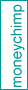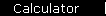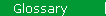# Compound Annual Growth Rate (CAGR)

Interest rate at which a given present value would "grow" to a given future value in a given amount of time. The formula is
CAGR   =   (FV/PV)1/n - 1

where FV is the future value, PV is the present value, and n is the number of years.

See the interactive graph for an explanation of the formula and some examples, or the CAGR calculator.

Also see the CAGR of the S&P 500.

 home  |  glossary  |  calculator  |  about us  |  books